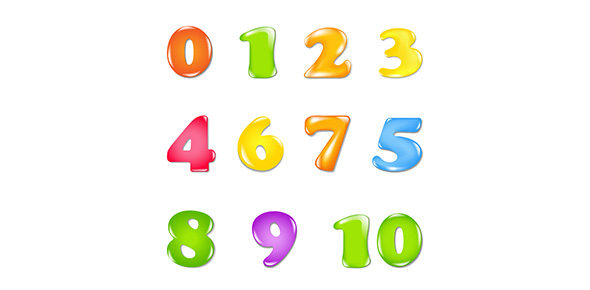# Greater Than, Less Than

Approved & Edited by ProProfs Editorial Team
The editorial team at ProProfs Quizzes consists of a select group of subject experts, trivia writers, and quiz masters who have authored over 10,000 quizzes taken by more than 100 million users. This team includes our in-house seasoned quiz moderators and subject matter experts. Our editorial experts, spread across the world, are rigorously trained using our comprehensive guidelines to ensure that you receive the highest quality quizzes.
| By Sarahshew
S
Sarahshew
Community Contributor
Quizzes Created: 1 | Total Attempts: 1,767
Questions: 6 | Attempts: 1,782SettingsThis quiz is to test your knowledge about which number is the greater or lesser number out of the two choices.

• 1.

### Which number is greater? 52 or 25

• A.

25

• B.

52

B. 52
Explanation
The number 52 is greater than 25 because 52 has a higher value.

Rate this question:

• 2.

### Using the greater than sign, which is greater?35___26

>
Explanation
The greater than sign (>) is used to compare two numbers and determine which one is greater. In this case, the number 35 is greater than 26.

Rate this question:

• 3.

### Which number is less than the other?42 or 68

• A.

68

• B.

42

B. 42
Explanation
The number 42 is less than the number 68.

Rate this question:

• 4.

### Using the correct sign, which is greater?48____ 72

<
Explanation
In the given question, we need to determine which number is greater between 48 and 72. To do this, we can compare the two numbers. Since 72 is greater than 48, the correct sign to use is the greater than sign (>), indicating that 72 is greater than 48.

Rate this question:

• 5.

### Which number is greater?97 or 102

• A.

97

• B.

102

B. 102
Explanation
The number 102 is greater than 97 because it has a higher value.

Rate this question:

• 6.

### Using the correct sign, which number is greater?13___81

<
Explanation
To determine which number is greater, we can compare the digits in the corresponding positions. The digit in the tens place of 13 is 1, while the digit in the tens place of 81 is 8. Since 8 is greater than 1, the number 81 is greater than 13.

Rate this question:

Related TopicsBack to top